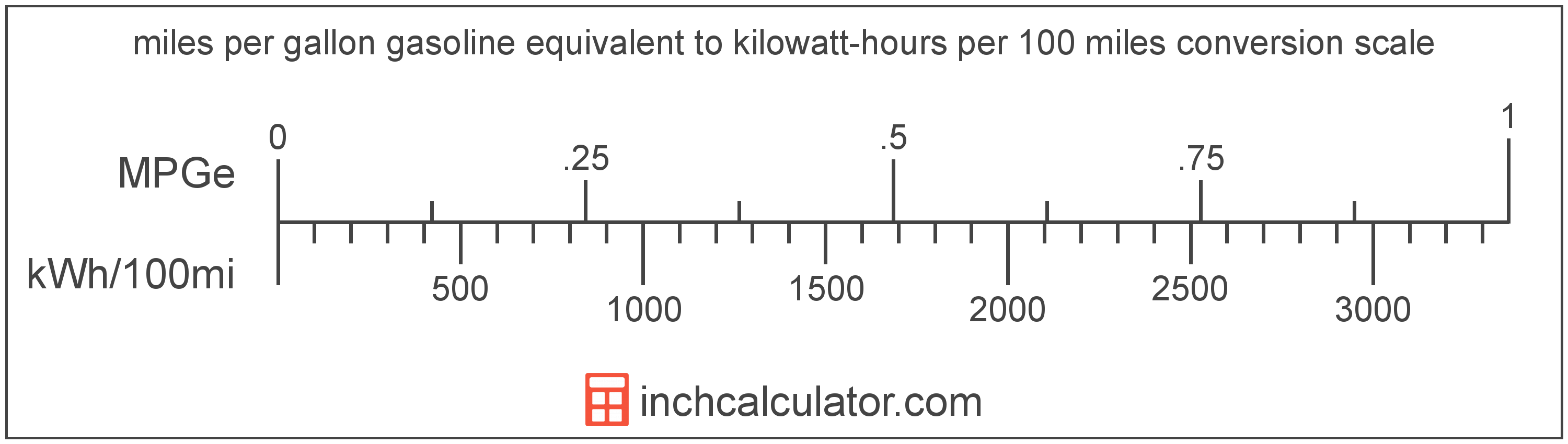# Kilowatt-Hours per 100 Miles to Miles per Gallon Gasoline Equivalent Converter (KWh/100mi to MPGe)

Enter the electric car efficiency in kilowatt-hours per 100 miles below to get the value converted to miles per gallon gasoline equivalent.

Results in Miles per Gallon Gasoline Equivalent:1 kWh/100mi = 3,370.5 MPGe

Do you want to convert MPGe to kWh/100mi?

## How to Convert Kilowatt-Hours per 100 Miles to Miles per Gallon Gasoline Equivalent

Convert kilowatt-hours per 100 miles to miles per gallon gasoline equivalent with this simple formula:

MPGe = 3370.5 ÷ kWh/100mi

Insert the kWh/100mi efficiency measurement in the formula and then solve to find the result.

For example, let's convert 50 kWh/100mi to MPGe:

50 kWh/100mi = ( 3370.5 ÷ 50 ) = 67.41 MPGeKilowatt-hours per 100 miles and miles per gallon gasoline equivalent are both units used to measure electric car efficiency. Keep reading to learn more about each unit of measure.

## What Are Kilowatt-Hours per 100 Miles?

Kilowatt-hours per 100 miles are a measure of electric vehicle efficiency measuring the kilowatt-hours of energy required to travel 100 miles.

The kilowatt-hour per 100 miles is a US customary unit of electric car efficiency. Kilowatt-hours per 100 miles can be abbreviated as kWh/100mi; for example, 1 kilowatt-hour per 100 miles can be written as 1 kWh/100mi.

In the expressions of units, the slash, or solidus (/), is used to express a change in one or more units relative to a change in one or more other units.

## What Are Miles per Gallon Gasoline Equivalent?

Miles per gallon gasoline equivalent is a way of measuring the energy needed equivalent to a gallon of gasoline to travel a distance in miles.

Because electric vehicles do not consume gasoline, the EPA set an equivalent amount of energy in kilowatt-hours that is equal to one gallon of gasoline. According to the EPA, one gallon of gasoline is equal to 33.705 kilowatt-hours.

The mile per gallon gasoline equivalent is a US customary unit of electric car efficiency. Miles per gallon gasoline equivalent can be abbreviated as MPGe; for example, 1 mile per gallon gasoline equivalent can be written as 1 MPGe.

## Kilowatt-Hour per 100 Miles to Mile per Gallon Gasoline Equivalent Conversion Table

Table showing various kilowatt-hour per 100 miles measurements converted to miles per gallon gasoline equivalent.
Kilowatt-hours Per 100 Miles Miles Per Gallon Gasoline Equivalent
0.001 kWh/100mi 3,370,500 MPGe
0.002 kWh/100mi 1,685,250 MPGe
0.003 kWh/100mi 1,123,500 MPGe
0.004 kWh/100mi 842,625 MPGe
0.005 kWh/100mi 674,100 MPGe
0.006 kWh/100mi 561,750 MPGe
0.007 kWh/100mi 481,500 MPGe
0.008 kWh/100mi 421,313 MPGe
0.009 kWh/100mi 374,500 MPGe
0.01 kWh/100mi 337,050 MPGe
0.02 kWh/100mi 168,525 MPGe
0.03 kWh/100mi 112,350 MPGe
0.04 kWh/100mi 84,263 MPGe
0.05 kWh/100mi 67,410 MPGe
0.06 kWh/100mi 56,175 MPGe
0.07 kWh/100mi 48,150 MPGe
0.08 kWh/100mi 42,131 MPGe
0.09 kWh/100mi 37,450 MPGe
0.1 kWh/100mi 33,705 MPGe
0.2 kWh/100mi 16,853 MPGe
0.3 kWh/100mi 11,235 MPGe
0.4 kWh/100mi 8,426 MPGe
0.5 kWh/100mi 6,741 MPGe
0.6 kWh/100mi 5,618 MPGe
0.7 kWh/100mi 4,815 MPGe
0.8 kWh/100mi 4,213 MPGe
0.9 kWh/100mi 3,745 MPGe
1 kWh/100mi 3,371 MPGe

## References

1. United States Environmental Protection Agency, Technology, https://www3.epa.gov/otaq/gvg/learn-more-technology.htm